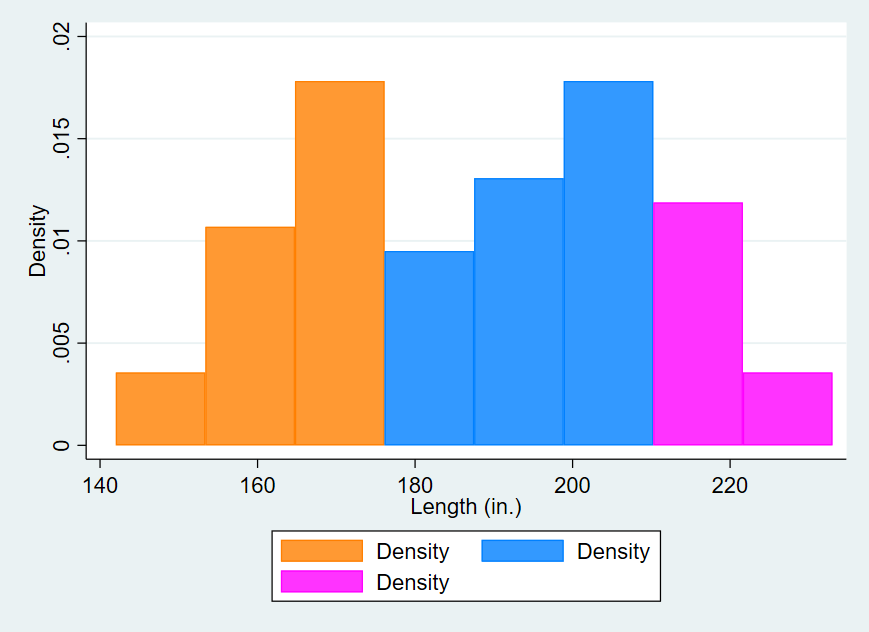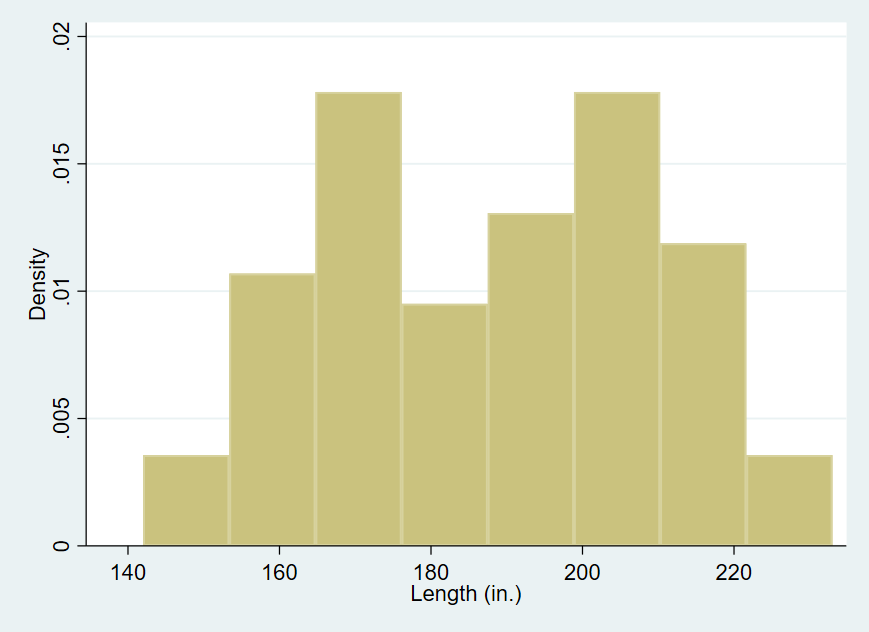Search

# Colour Block Your Histogram - Stata Quick Tip

This short tip will show you how to colour the bars of your histogram according to value ranges along the x-axis. This allows you to colour-code different regions of your x variable. This tip was originally posted on twitter by John Mullahy (@JohnMullahy). You can find the original tweet here. It uses a Stata subroutine of the histogram command to generate the y and x data needed to create the graph.

## How to Use:

note there are two underscores between twoway and histogram below

*Make a note of the bar width of your histogram, then

## Worked Example:

I will use the Stata auto example dataset to make a histogram of the variable length. I want to use the default Stata bar width, and there are two main ways for me to calculate this. First I can simply run a basic histogram with the command histogram length, and the pre-calculated width will be printed on the results screen. Alternatively I can calculate it by running summarize length, then use the minimum and maximum values to calculate the bar width for the standard 8 bars ((max – min)/8).

For this example I am going to use some local macros to get my bar width by the calculation outlined above. I will then separate my histogram into 3 separate bar sections. In the command pane I type the following:

This gives the following graph:Compare this to the original look of the histogram when run by the histogram command, below: